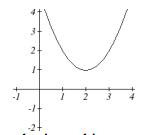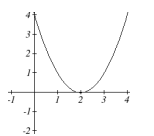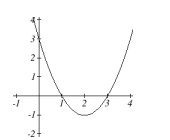## The Discriminant

### Learning Outcome

• Use the discriminant to classify solutions to quadratic equations

## The Discriminant

The quadratic formula not only generates the solutions to a quadratic equation, but can also tell us about the nature of the solutions. When we consider the discriminant, or the expression under the radical, ${b}^{2}-4ac$, it tells us whether the solutions are real numbers or complex numbers and how many solutions of each type to expect.

Let us explore how the discriminant affects the evaluation of $\sqrt{{{b}^{2}}-4ac}$ in the quadratic formula and how it helps to determine the solution set.

• If $b^{2}-4ac>0$, then the number underneath the radical will be a positive value. You can always find the square root of a positive number, so evaluating the quadratic formula will result in two real solutions (one by adding the positive square root and one by subtracting it).
• If $b^{2}-4ac=0$, then you will be taking the square root of $0$, which is $0$. Since adding and subtracting $0$ both give the same result, the “$\pm$” portion of the formula does not matter. There will be one real repeated solution.
• If $b^{2}-4ac<0$, then the number underneath the radical will be a negative value. Since you cannot find the square root of a negative number using real numbers, there are no real solutions. However, you can use imaginary numbers. You will then have two complex solutions, one by adding the imaginary square root and one by subtracting it.

The table below summarizes the relationship between the value of the discriminant and the solutions of a quadratic equation.

Value of Discriminant Results
${b}^{2}-4ac=0$ One repeated rational solution
${b}^{2}-4ac>0$, perfect square Two rational solutions
${b}^{2}-4ac>0$, not a perfect square Two irrational solutions
${b}^{2}-4ac<0$ Two complex solutions

### The Discriminant

For $a{x}^{2}+bx+c=0$, where $a$, $b$, and $c$ are real numbers, the discriminant is the expression under the radical in the quadratic formula: ${b}^{2}-4ac$. It tells us whether the solutions are real numbers or complex numbers and how many solutions of each type to expect.

### Example

Use the discriminant to determine how many and what kind of solutions the quadratic equation $x^{2}-4x+10=0$ has.

### Example

Use the discriminant to find the nature of the solutions to the following quadratic equations:

1. ${x}^{2}+4x+4=0$
2. $8{x}^{2}+14x+3=0$
3. $3{x}^{2}-5x - 2=0$
4. $3{x}^{2}-10x+15=0$

### Try It

In the last example, we will draw a correlation between the number and type of solutions to a quadratic equation of the form $0=ax^2+bx+c$ and its corresponding graph for $y=ax^2+bx+c$.  (Remember, the x-intercepts correspond to the solutions of the equation $0=ax^2+bx+c$).

### Example

Use the following graphs of equations of the form $y=ax^2+bx+c$ to determine how many and what type of solutions the corresponding quadratic equation $ax^2+bx+c=0$ will have.    Determine whether the discriminant will be greater than, less than, or equal to zero for each.

a.b.c.We can summarize our results as follows:

 Discriminant Number and Type of Solutions Graph of $y=ax^2+bx+c$ $b^{2}-4ac<0$ two complex solutions will not cross the x-axis $b^{2}-4ac=0$ one real repeated solution will touch x-axis once $b^{2}-4ac>0$ two real solutions will cross x-axis twice

In the following video, we show more examples of how to use the discriminant to describe the type of solutions of a quadratic equation.

## Summary

The discriminant of the quadratic formula is the quantity under the radical, ${{b}^{2}}-4ac$. It determines the number and the type of solutions that a quadratic equation has. If the discriminant is positive, there are $2$ real solutions. If it is $0$, there is $1$ real repeated solution. If the discriminant is negative, there are $2$ complex solutions (but no real solutions).

The discriminant can also tell us about the behavior of the graph of a quadratic equation in two variables.

## Contribute!

Did you have an idea for improving this content? We’d love your input.